# How to Extract multiple match Values into different Columns or Rows

This post explains that how to extract multiple match values from a range, then put the values into the different columns in excel. And how to extract multiple matches into the different rows.

In the previous post, we talked that how to get the position of the nth occurrence of a value in a column, and this pose will refer to it to extract all matched values firstly, then put all returned values into the different columns.

## Extract multiple match values into separate columns

If you want to fetch all matches from a range then put it into cells in different columns, you can use a combination with the INDEX function, the SMALL function, the IF function,  the ROW function and the COLUMNS function to create a new excel formula.

For example, if you want to get all member names belong to “excel” team in the range B3:D7, then separate it into the different columns, such as: E2, F2…you can use the following formula:

`=IFERROR(INDEX(\$D\$3:\$D\$7,SMALL(IF(\$C\$3:\$C\$7="excel",ROW(\$C\$3:\$C\$7)-ROW(INDEX(\$C\$3:\$C\$7,1,1))+1),COLUMNS(\$E\$2:E2))),"")`

Let’s see how the above formula works:

= ROW(\$C\$3:\$C\$7)-ROW(INDEX(\$C\$3:\$C\$7,1,1))+1

The ROW function returns the relative position of all rows in the range C3:C7.  The returned result is another array.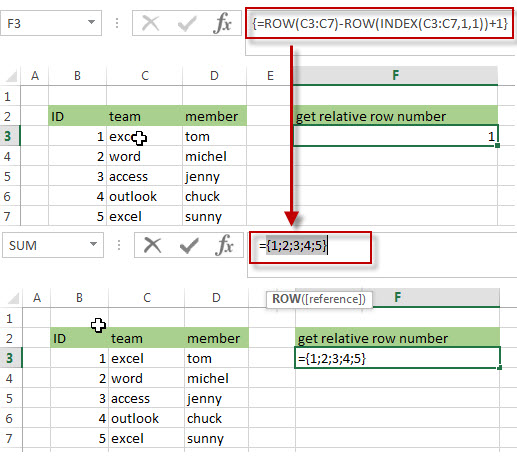= IF(\$C\$3:\$C\$7=”excel”,ROW(\$C\$3:\$C\$7)-ROW(INDEX(\$C\$3:\$C\$7,1,1))+1)

The IF function will check if each value in range C3:C7 is equal to “excel”, if TRUE, returns its relative position, otherwise, returns FALSE. As this formula is an array formula, so it must be returned an array result.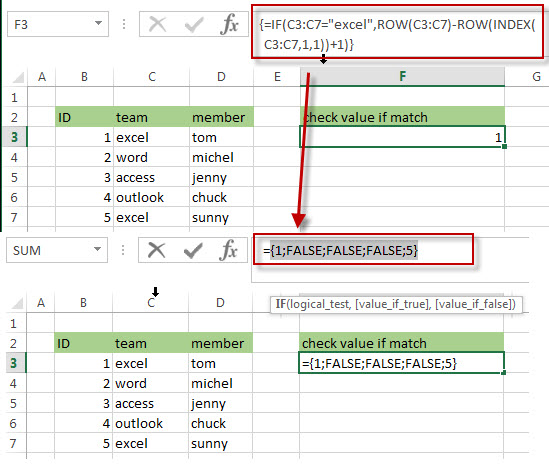=COLUMNS(\$E\$2:E2)

The COLUMNS function returns a numeric values, it will be used by the SMALL function as its nth argument to indicate the position of the number that you want to return.

=SMALL(IF(\$C\$3:\$C\$7=”excel”,ROW(\$C\$3:\$C\$7)-ROW(INDEX(\$C\$3:\$C\$7,1,1))+1),COLUMNS(\$E\$2:E2))

The SMALL function returns the position of the nth smallest values from the numbers in an array result returned by the above IF function. The nth argument is specified by the result returned by the COLUMNS function.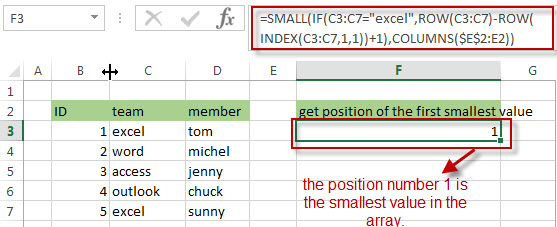=INDEX(\$D\$3:\$D\$7,SMALL(IF(\$C\$3:\$C\$7=”excel”,ROW(\$C\$3:\$C\$7)-ROW(INDEX(\$C\$3:\$C\$7,1,1))+1),COLUMNS(\$E\$2:E2)))

The INDEX function returns a value at a position returned by the above SMALL function in the range D3:D7, so it should be the first matches of the member name that belong to the excel team in the range B3:D7.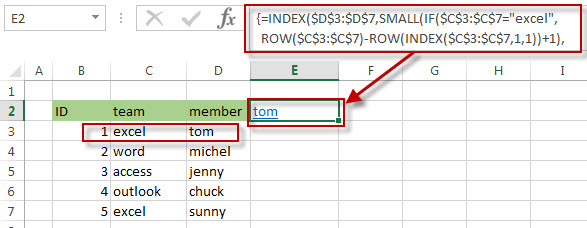And if you want to get the second matches into Cell F2, you just need to drag the fill hander in the Cell E2 to F2, then the second matches of the member name will be fetched in the Cell F2.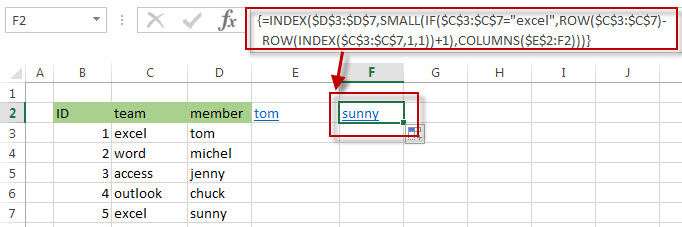=IFERROR()

The IFERROR function will check if the result returned by the SMALL function is a #NUM error, then returns an empty string.

## Extract multiple match values into separate rows

If you want to extract multiple matches into the separate rows in excel, just need to use ROWS instead of the COLUMNS function in the above formula as follows:

`=IFERROR(INDEX(\$D\$3:\$D\$7,SMALL(IF(\$C\$3:\$C\$7="excel",ROW(\$C\$3:\$C\$7)-ROW(INDEX(\$C\$3:\$C\$7,1,1))+1), ROWS(\$E\$2:E2))),"")`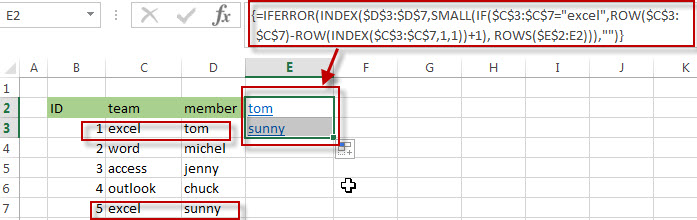If you want to extract the second matches, then put the result into the cell E3, you just need to drag the Fill Handler in Cell E2 to E3. The second matches of the member name should be fetched in the Cell E3.

### Related Formulas

• Get the Position of the nth Occurrence of a Character in a Cell
If you want to get the position of the nth occurrence of a character using a excel formula, you can use the FIND function in combination with the SUBSTITUTE function.
•  Get the position of Last Occurrence of a character or string in a cell
If you want to get the position of the last occurrence of a character in a cell, then you can use a combination of the LOOKUP function, the MID function, the ROW function, the INDIRECT function and the LEN function to create an excel formula.…
• Find the Relative Position in a Range or Table
If you want to know the relative row position for all rows in an Excel Range (B3:D6), you can use a excel Array formula as follows:=ROW(B3:D6)- ROW(B3) + 1. You can also use another excel array formula to get the same result as follows:=ROW(B3:D6)-ROW(INDEX(B3:D6,1,1))+1…
• Get the First Row Number in a Range
If the ROW function use a Range as its argument, it only returns the first row number.You can also use the ROW function within the MIN function to get the first row number in a range. You can also use the INDEX function to get the reference of the first row in a range, then combined to the ROW function to get the first row number of a range.…
•  Get the Last Row Number in a Range
If you want to get the last row number in a range, you need to know the first row number and the total rows number of a range, then perform the addition operation, then subtract 1, the last result is the last row number for that range.…
• Get the position of Last Occurrence of a value in a column
If you want to find the position number of the last occurrence of a specific value in a column (a single range), you can use an array formula with a combination of the MAX function, IF function, ROW function and INDEX Function.

### Related Functions

• Excel IFERROR function
The Excel IFERROR function returns an alternate value you specify if a formula results in an error, or returns the result of the formula.The syntax of the IFERROR function is as below:= IFERROR (value, value_if_error)…
• Excel SMALL function
The Excel SMALL function returns the smallest numeric value from the numbers that you provided. Or returns the smallest value in the array.The syntax of the SMALL function is as below:=SMALL(array,nth) …
• Excel INDEX function
The Excel INDEX function returns a value from a table based on the index (row number and column number)The INDEX function is a build-in function in Microsoft Excel and it is categorized as a Lookup and Reference Function.The syntax of the INDEX function is as below:= INDEX (array, row_num,[column_num])…
• Excel ROW function
The Excel ROW function returns the row number of a cell reference.The ROW function is a build-in function in Microsoft Excel and it is categorized as a Lookup and Reference Function.The syntax of the ROW function is as below:= ROW ([reference])….
• Excel MIN function
The Excel MIN function returns the smallest numeric value from the numbers that you provided. Or returns the smallest value in the array.The MIN function is a build-in function in Microsoft Excel and it is categorized as a Statistical Function.The syntax of the MIN function is as below:= MIN(num1,[num2,…numn])….
• Excel IF function
The Excel IF function perform a logical test to return one value if the condition is TRUE and return another value if the condition is FALSE. The IF function is a build-in function in Microsoft Excel and it is categorized as a Logical Function.The syntax of the IF function is as below:= IF (condition, [true_value], [false_value])….
• Excel ROWS function
The Excel ROWS function returns the number of rows in a cell reference.The ROWS function is a build-in function in Microsoft Excel and it is categorized as a Lookup and Reference Function.The syntax of the ROWS function is as below:= ROWS(array)….
• Excel COLUMNS function
The Excel COLUMNS function returns the number of columns in an Array or a reference.The syntax of the COLUMNS function is as below:=COLUMNS (array)….

Related Posts
How to Sum the Smallest N Values in Excel

Sometimes we may want to sum the first smallest N numbers in a range in Excel. In this article, we will show you the method of “SUM the Smallest N Numbers” by a simple formula which consist of SUMPRODUCT and ...

How to Create Dynamical Drop-Down List and Sort by Alphabetical Order in Excel

In our daily work we may need to create a dynamical dropdown list and sort all values by alphabetical order. To create a dropdown list like this, we need to apply some built-in features like ‘Define Name’ and ‘Data Validation’ ...

How to Find the Smallest Value and Smallest Positive Value in Excel

Sometimes we want to find out the smallest positive value among a set of values. We should ignore the negative value and zero value when getting the smallest positive value. So we design different cases in this article to demonstrate ...

How to Convert Multiple Rows into a Single Row in Excel

This post will guide you how to convert multiple rows or columns into a single row with a formula in Excel 3013/2016. How do I convert groups of rows to a single row of columns with VBA Macro in Excel. ...

How to Remove Numbers from Text in Excel

This post will guide you how to remove all numbers from a text string in one cell in Excel. How do I strip all numeric characters from a given cell with a formula in Excel. How do I remove numbers ...

How to Highlight Every Other Row Using Conditional Formatting in Excel

This post will guide you how to highlight every other row in Excel. How do I shade every other row with conditional formatting in Excel. Highlight Every Other Row Video: Highlight Every Other Row Highlight Every Other Row Assuming that ...

How to Get the First, 2nd or Nth Match Using Vlookup/Index/Match

This post will guide you how get the nth matching values with VLOOKUP function in Excel. How do I find the nth match value with Index/Match formula in Excel. How to find the first, second, third or nth matching value ...

Ignoring Blank or Zero Cells with Conditional formatting

This post will guide you how to make conditional formatting ignore blank cells or zero cell in Excel. How do I force blank cells or zero cells to be ignored in conditional formatting in Excel. How to ignore blank cells ...

Find the Earliest and Latest Date in a Range of Dates in Excel

This post will guide you how to find the earliest date in a range of dates in Excel. How do I get the earliest and latest date in a range with a formula in Excel. How to find the highest ...

Find Closest Value or Nearest Value in a Range in Excel

This post will guide you how to find the closest value or nearest value in a range of cells or in a column in Excel. How do I find the closest match in a range to a given lookup value ...

Sidebar